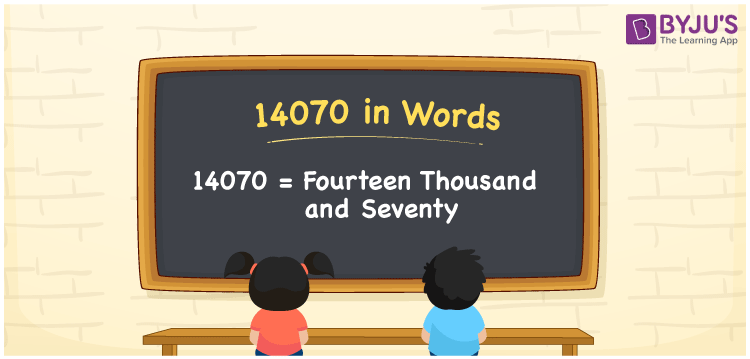# 14070 in words

14070 in words is written as Fourteen Thousand and Seventy. In 14070, 1 has a place value of ten thousand, 4 is in the place value of thousand and 7 is in the place value of ten. The article on Place Value gives more information. The number 14070 is used in expressions that relate to money, distance, social media views, and many more. For example, “Polling booth number 5 has Fourteen Thousand and Seventy voters in the list.”

 14070 in words Fourteen Thousand and Seventy Fourteen Thousand and Seventy in Numbers 14070

## 14070 in English Words## How to Write 14070 in Words?

We can convert 14070 to words using a place value chart. The number 14070 has 5 digits, so let’s make a chart that shows the place value up to 5 digits.

 Ten thousand Thousands Hundreds Tens Ones 1 4 0 7 0

Thus, we can write the expanded form as:

1 × Ten thousand + 4 × Thousand + 0 × Hundred + 7 × Ten + 0 × One

= 1 × 10000 + 4 × 1000 + 0 × 100 + 7 × 10 + 0 × 1

= 14070.

= Fourteen Thousand and Seventy.

14070 is the natural number that is succeeded by 14069 and preceded by 14071.

14070 in words – Fourteen Thousand and Seventy.

Is 14070 an odd number? – No.

Is 14070 an even number? – Yes.

Is 14070 a perfect square number? – No.

Is 14070 a perfect cube number? – No.

Is 14070 a prime number? – No.

Is 14070 a composite number? – Yes.

## Solved Example

1. Write the number 14070 in expanded form

Solution: 1 x 10000 + 4 x 1000 + 0 x 100 + 7 x 10 + 0 x 1

We can write 14070 = 10000 + 4000 + 000 + 70 + 0

= 1 x 10000 + 4 x 1000 + 0 x 100 + 7 x 10 + 0 x 1.

## Frequently Asked Questions on 14070 in words

Q1

### How to write the number 14070 in words?

14070 in words is written as Fourteen Thousand and Seventy.
Q2

### Is 14070 a prime number?

No. 14070 is not a prime number.
Q3

### Is 14070 divisible by 10?

Yes. 14070 is divisible by 10.